Sponsored Link •Weblogs Forum
The Cat Type Inference Algorithm

6 replies on 1 page. Most recent reply: Nov 13, 2006 12:09 PM by Christopher Diggins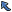Back to Topic List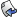Reply to this Topic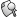Search Forum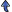Threaded View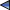Previous Topic Next Topic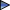Flat View: This topic has 6 replies on 1 page
 Christopher Diggins Posts: 1215 Nickname: cdiggins Registered: Feb, 2004
The Cat Type Inference Algorithm (View in Weblogs)
Posted: Nov 9, 2006 9:23 AMReply
Summary
I've posted an updated version of the Cat manual with a generalized description of the type inference algorithm used in Cat. This post contains an excerpt of the type inference algorithm description.
 AdvertisementHere is an excerpt of the current Cat manual as it pertains to the current Cat release, version 0.9.7. I have to apologize in advance, it is a bit dense, but it should be useful if you are interested in the nitty gritty of how type inference can work in a stack-based language.

## Type Checking and Type Inference

This section provides a description of the algorithm for checking well-typedness and inferring types of Cat functions.

The primary and auxiliary stacks in Cat can be viewed as a pair of tuples. Every function expresses a mapping from a pair of a tuples to another pair of tuples. We can express the consumption and production of a function type using the following short hand notation, where index 0 represents the type at the top of the stack.

```    primary consumption   = PC = (pc0, pc1, ..., pcN)
auxiliary consumption = AC = (ac0, ac1, ..., acN)
primary production    = PP = (pp0, pc1, ..., pcN)
auxiliary production  = AP = (ap0, ap1, ..., apN)
```
From this notation the types of two functions can be expressed as:
```    f : (PC(f), AC(f)) -> (PP(f), AP(f))
g : (PC(g), AC(g)) -> (PP(g), AP(g))
```
The superset relation of tuples as follows:
```    (a0, a1, ..., aN) >= (b0, b1, ..., bM)
iff (N > M) and forall i=0 to M (ai = b0)
```
A Cat function is well typed according to the following function definition:
```    welltyped(f . g) iff
PC(g) >= PP(f) or PP(f) >= PC(g)
```
Consider the following definitions of addition and subtraction:
```    (a0, a1, ..., aN) + (b0, b1, ..., bM)
= (a0, a1, ..., aN, b0, b1, ..., bM)

(a0, a1, ..., aN) - (b0, b1, ..., bM)
= M > N | (b[N+1], b[N+2], ..., bM)
M <= N | ()
```
Using these definition we can define the consumption and production of the compose of the two functions as follows:
```    PC(f . g) = PC(f) + (PC(g) - PP(f))
AC(f . g) = AC(f) + (AC(g) - AP(f))
PP(f . g) = PP(g) + (PP(f) - PC(g))
AP(f . g) = AP(g) + (AP(f) - AC(g))
```
Putting this together the type of two consecutive functions is:
```    f . g : (PC(f) + (PC(g) - PP(f)), AC(f) + (AC(g) - AP(f)))
-> (PP(g) + (PP(f) - PC(g)), AP(g) + (AP(f) - AC(g)))
```

That is all there is to it. I know it's a bit dense, but hopefully it can still be of some use. If you have some suggestions on how I can make it more clear, and use more standardized language or notation I would be very appreciative. Even if you just point out the confusing bits, that would be very helpful!

 Reno C. Posts: 10 Nickname: saxml Registered: Oct, 2005
Re: The Cat Type Inference Algorithm Posted: Nov 11, 2006 7:16 PMReply
My apologies if I screw up everything but I think you made some mistakes in you definitions and assertions.

> The superset relation of tuples as follows:
> <pre>
> (a0, a1, ..., aN) >= (b0, b1, ..., bM)
> iff (N > M) and forall i=0 to M (ai = b0)
> </pre>

Shouldn't it be indeed:
`     (a0, a1, ..., aN) >= (b0, b1, ..., bM)     iff (N >= M) and forall i=0 to M (ai = bi)`
?

> A Cat function is well typed according to the following
> function definition:
>
> <pre>
> welltyped(f . g) iff
> PC(g) >= PP(f) or PP(f) >= PC(g)
> </pre>

It would mean PC(g) >= PP(f) >= PC(g)

?

Is it possible to define welltyped(f . g) without considering the state of the stack(s) ?
I don't think so ! (again, all my apologies if I'm totaly wrong ;)
A new definition:
`     Let T be a tuple representing the primary stack     welltyped(f . g) iff        T >= PC(g) and         T - PC(g) + PP(g) >= PC(f)`

> Using these definition we can define the consumption
> and production of the compose of the two functions as
> as follows:
>
> <pre>
> PC(f . g) = PC(f) + (PC(g) - PP(f))
> AC(f . g) = AC(f) + (AC(g) - AP(f))
> PP(f . g) = PP(g) + (PP(f) - PC(g))
> AP(f . g) = AP(g) + (AP(f) - AC(g))
> </pre>
>

I don't get it! I tried with Cat 0.9.7 and it seems to me that the relations are (only for the primary stack):

`     PC(f . g) = max(PC(g), PC(g) + (PC(f) - PP(g)))     PP(f . g) = max((), (PP(g) - PC(f)) + PP(f))`

() being the empty tuple

 Christopher Diggins Posts: 1215 Nickname: cdiggins Registered: Feb, 2004
Re: The Cat Type Inference Algorithm Posted: Nov 12, 2006 8:24 AMReply
> My apologies if I screw up everything but I think you made
> some mistakes in you definitions and assertions.
>
> > The superset relation of tuples as follows:
> > <pre>
> > (a0, a1, ..., aN) >= (b0, b1, ..., bM)
> > iff (N > M) and forall i=0 to M (ai = b0)
> > </pre>
>
> Shouldn't it be indeed:
>
`     (a0, a1, ..., aN) >= (b0, b1, ..., bM)>      iff (N >= M) and forall i=0 to M (ai = bi)> `
?

Yes!

> > A Cat function is well typed according to the
> following
> > function definition:
> >
> > <pre>
> > welltyped(f . g) iff
> > PC(g) >= PP(f) or PP(f) >= PC(g)
> > </pre>
>
> It would mean PC(g) >= PP(f) >= PC(g)
>
> ?

I believe your deduction would be correct if I used "and" instead of "or". Do you agree?

> Is it possible to define welltyped(f . g) without
> considering the state of the stack(s) ?
> I don't think so ! (again, all my apologies if I'm totaly
> wrong ;)

That is an excellent point. You can create a wellformed polymorphic function, but it is not type complete. Therefore calling it welltyped is a mistake!

> A new definition:
>
`     Let T be a tuple representing the primary stack>      welltyped(f . g) iff >        T >= PC(g) and  >        T - PC(g) + PP(g) >= PC(f)> `

Sweet!

> > Using these definition we can define the consumption
> > and production of the compose of the two functions as
> > as follows:
> >
> > <pre>
> > PC(f . g) = PC(f) + (PC(g) - PP(f))
> > AC(f . g) = AC(f) + (AC(g) - AP(f))
> > PP(f . g) = PP(g) + (PP(f) - PC(g))
> > AP(f . g) = AP(g) + (AP(f) - AC(g))
> > </pre>
> >
>
> I don't get it! I tried with Cat 0.9.7 and it seems to me
> that the relations are (only for the primary stack):
>
>
`     PC(f . g) = max(PC(g), PC(g) + (PC(f) - PP(g)))>      PP(f . g) = max((), (PP(g) - PC(f)) + PP(f))> `

> () being the empty tuple

LOL! That would explain a few bugs I'm seeing! I believe the interpreter is flawed. This definitely convinces me that I need to rewrite the inference engine before releasing the version 1.

Thank you very much for taking the time to go over this and point out my problems. If I may ask what your full name is so that I can acknowledging your contribution? I'd like to talk to you on email if possible as well, my email is cdiggins @ gmail . com.

Thanks again!

 Christopher Diggins Posts: 1215 Nickname: cdiggins Registered: Feb, 2004
Re: The Cat Type Inference Algorithm Posted: Nov 12, 2006 8:47 AMReply
> I don't get it! I tried with Cat 0.9.7 and it seems to me
> that the relations are (only for the primary stack):
>
>
`     PC(f . g) = max(PC(g), PC(g) + (PC(f) - PP(g)))>      PP(f . g) = max((), (PP(g) - PC(f)) + PP(f))> `

> () being the empty tuple

This seems a bit weird, I don't know how the algorithm could be so wrong. I just want to make sure of something, you are aware of the recent decision I made to reverse the stack notation? e.g.

`define f { 5 true } `

Now has the following type:

`f : ()->(bool int)`

`f : ()->(int bool)`

 Reno C. Posts: 10 Nickname: saxml Registered: Oct, 2005
Re: The Cat Type Inference Algorithm Posted: Nov 12, 2006 11:36 AMReply
> > It would mean PC(g) >= PP(f) >= PC(g)
> >
> > ?

> I believe your deduction would be correct if I used "and" instead of "or". Do you agree?

I thought your "or" was the logical disjonction (different than the exclusive disjonction "xor").
Then both can be true at the same time (and your superset relation ">=" is reflexive). Anyway I don't understand your initial welltyped(f . g) definition

> you are aware of the recent decision I made to reverse the stack notation?

Not really. Anyway I noticed some examples in the Cat manual do not reflect what is implemented in Cat 0.9.7. For example in the manual there is:

`    define g { swap dup }    Will have it's type inferred by the compiler as:    g : (A:any B:any)->(B B A)`

In Cat 0.9.7 the type is (A:any B:any)->(B A A) which seems correct.

Reversing the stack do not have an impact on the definitions with tuples we wrote (well, i "guess"). But it is a good choice as well because the stack(s) will have the same order than the standard tuples notations (a0, .., aN) instead of (aN, .. a0). Is it the reason of your decision ?

I emailed you

Thank you

 Reno C. Posts: 10 Nickname: saxml Registered: Oct, 2005
Re: The Cat Type Inference Algorithm Posted: Nov 12, 2006 3:00 PMReply
A remark:

`PP(f . g) = max((), (PP(g) - PC(f)) + PP(f))`

max((), T) seems weird since T >= () is always true.
I inserted the max() in my first post because I was about to rewrite the subtraction definition for our tuples but I dropped it.

It was:

`    let Ta be a tuple (a0, a1, ..., aN)    let Tb be a tuple (b0, b1, ..., bM)        when N >= M, Ta - Tb = (a[M+1], ..., aN)    when N < M, Ta - Tb is not defined`

"not defined" then a need for the max()
In practice, if a Cat program encounters such a not defined subtraction, it means the program is not welltyped. Don't you think so ?

 Christopher Diggins Posts: 1215 Nickname: cdiggins Registered: Feb, 2004
Re: The Cat Type Inference Algorithm Posted: Nov 13, 2006 12:09 PMReply
> "not defined" then a need for the max()
> In practice, if a Cat program encounters such a not
> defined subtraction, it means the program is not
> welltyped. Don't you think so ?

I felt the definition was neccessary for constructing the rules about typing.

However you've helped me identify some serious weaknesses in my current rationalization of the type sytem. For that I am very grateful!

The next release of Cat will have a signficantly more improved type system and should support the notion of subtyping. I would like to resume the discussion when I make a new release.

 Flat View: This topic has 6 replies on 1 pagePrevious Topic Next TopicWeb Artima.com